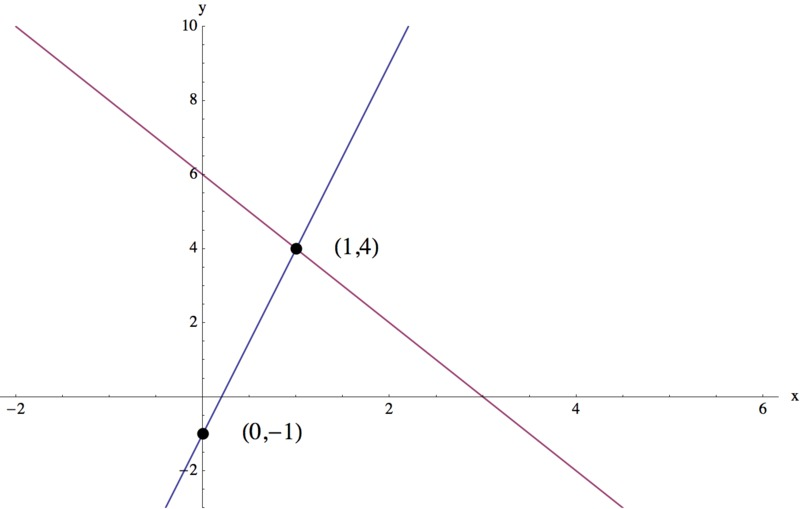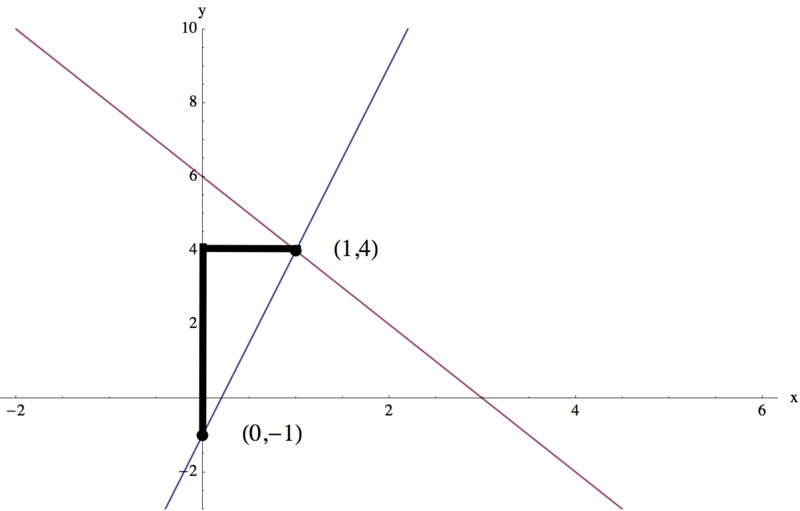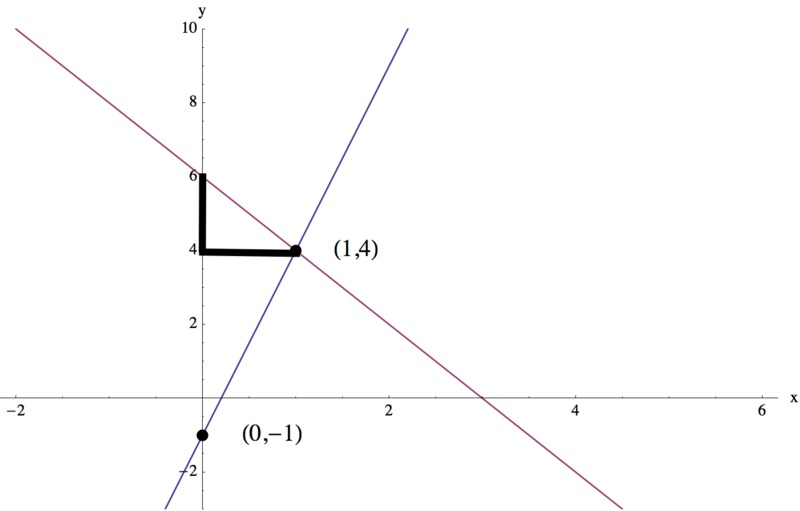# The Intersection of Two Lines

Alignments to Content Standards: 8.EE.C.8.a

1. Draw the two lines that intersect only at the point $(1,4)$. One of the lines should pass through the point $(0,-1)$.
2. Write the equation of each of the lines you created in part (a).
3. If we consider two or more equations together we have a system of equations. A solution to a system of equations in $x$ and $y$ is a pair of values $a$ and $b$ for $x$ and $y$ that make all of the equations true.

Do you think such a solution exists for the system of equations in part (b)? Explain.

4. Can you determine whether a system of equations has a solution by looking at the graph of the equations? Explain.

## IM Commentary

The purpose of this task is to introduce students to systems of equations. It takes skills and concepts that students know up to this point, such as writing the equation of a given line, and uses it to introduce the idea that the solution to a system of equations is the point where the graphs of the equations intersect (assuming they do). This task does not delve deeply into how to find the solution to a system of equations because it focuses more on the student's comparison between the graph and the system of equations.

The language in the task stem states that a solution to a system of equations is a pair of values that make all of the equations true. The solution shortens this to "satisfying" the equations--this is a more succinct way of saying it, but students may not know that "the ordered pair of values $(a,b)$ satisfies an equation" means "$a$ and $b$ make the equation true when $a$ is substituted for $x$ and $b$ is substituted for $y$ in the equation." Many people, books, and assessments talk about pairs of values "satisfying" an equation, so it would be helpful to students to have the meaning of this word made explicit.

## Solution

1. Constructing a set of axes, we can first locate the two given points, $(1,4)$ and $(0,-1)$, to create our first line. Our second line can be any other line that passes through $(1,4)$ but not $(0,-1)$, so there are many possible answers. Below is one possible construction:2. Focusing first on the line through the two given points, we can find the slope of this line two ways:

Graphically, we can start at the point $(0,-1)$ and then count how many units we go up divided by how many units we then go right to get to the point $(1,4)$, as in the diagram below.This gives a slope of $m=\displaystyle\frac{5}{1}=5$.

Algebraically, we can find the difference between the $y$-coordinates of the two points, and divide it by the difference between the $x$-coordinates. $$m=\frac{4-(-1)}{1-0}=5.$$ Because the $y$-intercept of this line is -1, we have $b=-1$. So, the equation of our first line is $y=5x-1$.

We can reason in a similar way for our second line. Graphically, we see our second line contains the point $(0,6)$, so we can start at the point $(0,6)$ and then count how many units we go down divided by how many units we then go right to get to the point $(1,4)$, as in the diagram below.This gives a slope of $\displaystyle m=\frac{-2}{1}=-2$.

We can also find the slope algebraically: $$m=\frac{4-6}{1-0}=-2.$$ Because we have a $y$-intercept of 6, $b=6$. So, the equation of our first line is $y=-2x+6$.

3. Using this idea that a solution to a system of equations is a pair of values that makes both equations true, we decide that our system of equations does have a solution, because

• The coordinates of every point on a line satisfy its equation, and
• The point $(1,4)$ lies on both lines.

We can confirm that $(1,4)$ is our system's solution by substituting $x=1$ and $y=4$ into both equations: $$4=5(1)-1$$ and $$4=-2(1)+6.$$

4. Based on our work above, we can make a general observation that if a system of linear equations has a solution, that solution corresponds to the intersection point of the two lines because the coordinate pair naming every point on a graph is a solution to its corresponding equation.